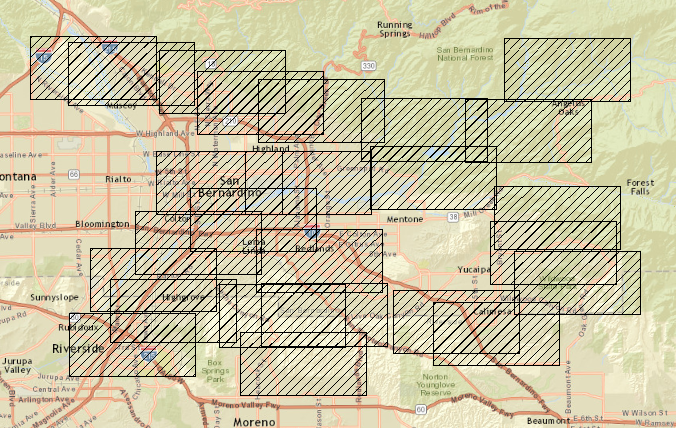# Polygon with given length and width JLF

242
2
10-11-2017 01:45 PMNew Contributor

How to create polygon (Projected NAD83) with specific length and width, but no X,Y points*

Tags (1)
2 RepliesbyMVP Esteemed Contributor
• Projected into what?
• NAD83 is a datum not a projection
• It can be done if you don't care where it is.

Perhaps you have other details that you could share.byEsri Esteemed Contributor

I totally agree with Dan.

It can be done, but a little bit more info would be appreciated. To create a rectangle you will need coordinates. You can create them randomly but you will still need some range of valid values. See below a sample script that will create a number of rectangles. In this case I took the projected coordinate system with NAD 1983 as datum and defined an extent for values values for xmin and ymin of the rectangles to be created:

``````def main():
import arcpy
import random
arcpy.env.overwriteOutput = True

# some settings
fc_out = r'C:\GeoNet\RandomRectangle\test2.shp'
width = 10000
height = 5000
xy_extent = arcpy.Extent(2048900, 549400, 2094700, 573600)
number_of_rectangles = 25

sr_pcs = arcpy.SpatialReference(103001)

feats = []
for i in range(number_of_rectangles):
# get a random xmin and ymin of the rectangle
xmin = random.randrange(xy_extent.XMin, xy_extent.XMax)
ymin =random.randrange(xy_extent.YMin, xy_extent.YMax)

# create the rectangle
pnts = [[xmin, ymin],
[xmin, ymin + height],
[xmin + width, ymin + height],
[xmin + width, ymin],
[xmin, ymin]]

polygon = arcpy.Polygon(arcpy.Array([arcpy.Point(*pnt) for pnt in pnts]), sr_pcs)
feats.append(polygon)

# write the rectangle to the output fc
arcpy.CopyFeatures_management(feats, fc_out)

if __name__ == '__main__':
main()‍‍‍‍‍‍‍‍‍‍‍‍‍‍‍‍‍‍‍‍‍‍‍‍‍‍‍‍‍‍‍‍‍‍‍‍``````

And the result:Could you elaborate a little more on what you are trying to achieve?